# Data Link Layer

DLL purpose? The goal of the data link layer is to provide reliable, efficient communication between adjacent machines connected by a single communication channel. Specifically:

1. Group the physical layer bit stream into units called frames. Note that frames are nothing more than ``packets'' or ``messages''. By convention, we'll use the term ``frames'' when discussing DLL packets.

2. Sender checksums the frame and sends checksum together with data. The checksum allows the receiver to determine when a frame has been damaged in transit.

3. Receiver recomputes the checksum and compares it with the received value. If they differ, an error has occurred and the frame is discarded.

4. Perhaps return a positive or negative acknowledgment to the sender. A positive acknowledgment indicate the frame was received without errors, while a negative acknowledgment indicates the opposite.

5. Flow control. Prevent a fast sender from overwhelming a slower receiver. For example, a supercomputer can easily generate data faster than a PC can consume it.

6. In general, provide service to the network layer. The network layer wants to be able to send packets to its neighbors without worrying about the details of getting it there in one piece.

At least, the above is what the OSI reference model suggests. As we will see later, not everyone agrees that the data link layer should perform all these tasks.

## Design Issues

If we don't follow the OSI reference model as gospel, we can imagine providing several alternative service semantics:

Reliable Delivery:
Frames are delivered to the receiver reliably and in the same order as generated by the sender.

Connection state keeps track of sending order and which frames require retransmission. For example, receiver state includes which frames have been received, which ones have not, etc.

Best Effort:
The receiver does not return acknowledgments to the sender, so the sender has no way of knowing if a frame has been successfully delivered.

When would such a service be appropriate?

1. When higher layers can recover from errors with little loss in performance. That is, when errors are so infrequent that there is little to be gained by the data link layer performing the recovery. It is just as easy to have higher layers deal with occasional lost packet.

2. For real-time applications requiring ``better never than late'' semantics. Old data may be worse than no data. For example, should an airplane bother calculating the proper wing flap angle using old altitude and wind speed data when newer data is already available?
Acknowledged Delivery:
The receiver returns an acknowledgment frame to the sender indicating that a data frame was properly received. This sits somewhere between the other two in that the sender keeps connection state, but may not necessarily retransmit unacknowledged frames. Likewise, the receiver may hand received packets to higher layers in the order in which the arrive, regardless of the original sending order.

Typically, each frame is assigned a unique sequence number, which the receiver returns in an acknowledgment frame to indicate which frame the ACK refers to. The sender must retransmit unacknowledged (e.g., lost or damaged) frames.

## Framing

The DLL translates the physical layer's raw bit stream into discrete units (messages) called frames. How can the receiver detect frame boundaries? That is, how can the receiver recognize the start and end of a frame?

Length Count:
Make the first field in the frame's header be the length of the frame. That way the receiver knows how big the current frame is and can determine where the next frame ends.

Disadvantage: Receiver loses synchronization when bits become garbled. If the bits in the count become corrupted during transmission, the receiver will think that the frame contains fewer (or more) bits than it actually does. Although checksum will detect the incorrect frames, the receiver will have difficulty resynchronizing to the start of a new frame. This technique is not used anymore, since better techniques are available.

Bit Stuffing:
Use reserved bit patterns to indicate the start and end of a frame. For instance, use the 4-bit sequence of 0111 to delimit consecutive frames. A frame consists of everything between two delimiters.

Problem: What happens if the reserved delimiter happens to appear in the frame itself? If we don't remove it from the data, the receiver will think that the incoming frame is actually two smaller frames!

Solution: Use bit stuffing. Within the frame, replace every occurrence of two consecutive 1's with 110. E.g., append a zero bit after each pair of 1's in the data. This prevents 3 consecutive 1's from ever appearing in the frame.

Likewise, the receiver converts two consecutive 1's followed by a 0 into two 1's, but recognizes the 0111 sequence as the end of the frame.

Example: The frame ``1011101'' would be transmitted over the physical layer as ``0111101101010111''.

Note: When using bit stuffing, locating the start/end of a frame is easy, even when frames are damaged. The receiver simply scans arriving data for the reserved patterns. Moreover, the receiver will resynchronize quickly with the sender as to where frames begin and end, even when bits in the frame get garbled.

The main disadvantage with bit stuffing is the insertion of additional bits into the data stream, wasting bandwidth. How much expansion? The precise amount depends on the frequency in which the reserved patterns appear as user data.

Character stuffing:
Same idea as bit-stuffing, but operates on bytes instead of bits.

Use reserved characters to indicate the start and end of a frame. For instance, use the two-character sequence DLE STX (Data-Link Escape, Start of TeXt) to signal the beginning of a frame, and the sequence DLE ETX (End of TeXt) to flag the frame's end.

Problem: What happens if the two-character sequence DLE ETX happens to appear in the frame itself?

Solution: Use character stuffing; within the frame, replace every occurrence of DLE with the two-character sequence DLE DLE. The receiver reverses the processes, replacing every occurrence of DLE DLE with a single DLE.

Example: If the frame contained ``A B DLE D E DLE'', the characters transmitted over the channel would be ``DLE STX A B DLE DLE D E DLE DLE DLE ETX''.

Disadvantage: character is the smallest unit that can be operated on; not all architectures are byte oriented.

Encoding Violations:
Send an signal that doesn't conform to any legal bit representation. In Manchester encoding, for instance, 1-bits are represented by a high-low sequence, and 0-bits by low-high sequences. The start/end of a frame could be represented by the signal low-low or high-high.

The advantage of encoding violations is that no extra bandwidth is required as in bit-stuffing. The IEEE 802.4 standard uses this approach.

Finally, some systems use a combination of these techniques. IEEE 802.3, for instance, has both a length field and special frame start and frame end patterns.

## Error Control

Error control is concerned with insuring that all frames are eventually delivered (possibly in order) to a destination. How? Three items are required.

Acknowledgements:
Typically, reliable delivery is achieved using the ``acknowledgments with retransmission'' paradigm, whereby the receiver returns a special acknowledgment (ACK) frame to the sender indicating the correct receipt of a frame.

In some systems, the receiver also returns a negative acknowledgment (NACK) for incorrectly-received frames. This is nothing more than a hint to the sender so that it can retransmit a frame right away without waiting for a timer to expire.

Timers:
One problem that simple ACK/NACK schemes fail to address is recovering from a frame that is lost, and as a result, fails to solicit an ACK or NACK. What happens if an ACK or NACK becomes lost?

Retransmission timers are used to resend frames that don't produce an ACK. When sending a frame, schedule a timer to expire at some time after the ACK should have been returned. If the timer goes off, retransmit the frame.

Sequence Numbers:
Retransmissions introduce the possibility of duplicate frames. To suppress duplicates, add sequence numbers to each frame, so that a receiver can distinguish between new frames and old copies.

## Flow Control

Flow control deals with throttling the speed of the sender to match that of the receiver. Usually, this is a dynamic process, as the receiving speed depends on such changing factors as the load, and availability of buffer space.

One solution is to have the receiver extend credits to the sender. For each credit, the sender may send one frame. Thus, the receiver controls the transmission rate by handing out credits.

In some cases, the data link layer service must be ``opened'' before use:

• The data link layer uses open operations for allocating buffer space, control blocks, agreeing on the maximum message size, etc.

• Synchronize and initialize send and receive sequence numbers with its peer at the other end of the communications channel.

## Error Detection and Correction

In data communication, line noise is a fact of life (e.g., signal attenuation, natural phenomenon such as lightning, and the telephone repairman). Moreover, noise usually occurs as bursts rather than independent, single bit errors. For example, a burst of lightning will affect a set of bits for a short time after the lightning strike.

Detecting and correcting errors requires redundancy -- sending additional information along with the data.

There are two types of attacks against errors:

Error Detecting Codes:
Include enough redundancy bits to detect errors and use ACKs and retransmissions to recover from the errors.
Error Correcting Codes:
Include enough redundancy to detect and correct errors.

To understand errors, consider the following:

1. Messages (frames) consist of m data (message) bits and r redundancy bits, yielding an n = (m+r)-bit codeword.

2. Hamming Distance. Given any two codewords, we can determine how many of the bits differ. Simply exclusive or (XOR) the two words, and count the number of 1 bits in the result.

3. Significance? If two codewords are d bits apart, d errors are required to convert one to the other.

4. A code's Hamming Distance is defined as the minimum Hamming Distance between any two of its legal codewords (from all possible codewords).

5. In general, all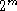possible data words are legal. However, by choosing check bits carefully, the resulting codewords will have a large Hamming Distance. The larger the Hamming distance, the better able the code can detect errors.

To detect d 1-bit errors requires having a Hamming Distance of at least d+1 bits. Why?

To correct d errors requires 2d+1 bits. Intuitively, after d errors, the garbled messages is still closer to the original message than any other legal codeword.

### Parity Bits

For example, consider parity: A single parity bit is appended to each data block (e.g. each character in ASCII systems) so that the number of 1 bits always adds up to an even (odd) number.

1000000(1) 1111101(0)

The Hamming Distance for parity is 2, and it cannot correct even single-bit errors (but can detect single-bit errors).

As another example, consider a 10-bit code used to represent 4 possible values: ``00000 00000'', ``00000 11111'', ``11111 00000'', and ``11111 11111''. Its Hamming distance is 5, and we can correct 2 single-bit errors:

For instance, ``10111 00010'' becomes ``11111 00000'' by changing only two bits.

However, if the sender transmits ``11111 00000'' and the receiver sees ``00011 00000'', the receiver will not correct the error properly.

Finally, in this example we are guaranteed to catch all 2-bit errors, but we might do better: if ``00111 00111'' contains 4 single-bit errors, we will reconstruct the block correctly.

### Single-Bit Error Correction

What's the fewest number of bits needed to correct single bit errors? Let us design a code containing n=m+r bits that corrects all single-bit errors (remember m is number of message (data) bits and r is number of redundant (check) bits):

1. There arelegal messages (e.g., legal bit patterns).

2. Each of the m messages has n illegal codewords a distance of 1 from it.That is, we systematically invert each bit in the corresponding n-bit codeword, we get n illegal codewords a distance of 1 from the original.

Thus, each message requires n+1 bits dedicated to it (n that are one bit away and 1 that is the message).

3. The total number of bit patterns =. That is, all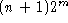encoded messages should be unique, and there can't be fewer messages than the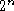possible codewords.

4. Since n=m+r, we get:, or.

This formula gives the absolute lower limit on the number of bits required to detect (and correct!) 1-bit errors.

Hamming developed a code that meets this lower limit:

• Bits are numbered left-to-right starting at 1.

• Bit numbers that are powers of two (e.g., 1, 2, 4, 8, etc.) are check bits; the remaining bits are the actual data bits.

• Each check bit acts as a parity bit for a set of bits (both data and check).

• To determine which parity bits in the codeword cover bit k of the codeword, rewrite bit position k as the a sum of powers of two (e.g., 19 = 1+2+16). A bit is checked by only those check bits in the expansion (e.g., check bits 1, 2, and 16).

• When a codeword arrives, examine each check bit k to verify that it has the correct parity. If not, add k to a counter. At the end of the process, a zero counter means no errors have occurred; otherwise, the counter gives the bit position of the incorrect bit.

For instance, consider the ascii character ``a'' = ``1100001''.

We know that:

• check bit 1 covers all odd numbered bits (e.g, 1, 3, 5,)

• check bit 2 covers bits 2, 3, 6, 7, 10, 11,• check bit 3 covers bits 4, 5, 6, 7, 12, 13, 14, 15,• check bit 4 covers bits 8, 9, 10, 11, 12, etc.

Thus:

• check bit 1 equals: ?+1+1+0+0+1 = 1

• check bit 2 equals: ?+1+0+0+0+1 = 0

• check bit 3 equals: ?+1+0+0 = 1

• check bit 4 equals: ?+0+0+1 = 1

giving:Note: Hamming Codes correct only single bit errors. To correct burst errors, we can send b blocks, distributing the burst over each of the b blocks.

For instance, build a b-row matrix, where each row is one block. When actually sending the data, send it one column at a time. If a burst error occurs, each block (row) will see a fraction of the errors, and may be able to correct its block.

Error correction is most useful in three contexts:

1. Simplex links (e.g., those that provide only one-way communication).

2. Long delay paths, where retransmitting data leads to long delays (e.g., satellites).

3. Links with very high error rates, where there is often one or two errors in each frame. Without forward error correction, most frames would be damaged, and retransmitting them would result in the frames becoming garbled again.

## Error Detection

Error correction is relatively expensive (computationally and in bandwidth).

For example, 10 redundancy bits are required to correct 1 single-bit error in a 1000-bit message. Detection? In contrast, detecting a single bit error requires only a single-bit, no matter how large the message.

The most popular error detection codes are based on polynomial codes or cyclic redundancy codes (CRCs).

Allows us to acknowledge correctly received frames and to discard incorrect ones.

## CRC Checksums

The most popular error detection codes are based on polynomial codes or cyclic redundancy codes. Idea:

• Represent a k-bit frame as coefficients of a polynomial expansion ranging from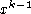to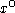, with the high-order bit corresponding to the coefficient of.

For example, represent the string ``11011'' as the polynomial: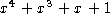• Perform modulo 2 arithmetic (e.g. XOR of the bits)

• Sender and receiver agree on a generator polynomial: G(x). (G(x) must be smaller than the number of bits in the message.)

• Append a checksum to message; let's call the message M(x), and the combination T(x). The checksum is computed as follows:

1. Let r be the degree of G(x), append r zeros to M(x). Our new polynomial becomes2. Divideby G(x) using modulo 2 arithmetic.

3. Subtract the remainder fromgiving us T(x).

• When receiver gets T(x), it divides T(x) by G(x); if T(x) divides cleanly (e.g., no remainder), no error has occurred.

The presence of a remainder indicates an error. What sort of errors will we catch?

Assume:

• the receiver gets T(x) + E(x), where each bit in E(x) corresponds to an error bit.

• k 1 bits indicate k single-bit errors.

• Receiver computes [T(x) + E(x)]/G(x) = E(x)/G(x).

Will detect:

• single bit errors. If a single-bit error occurs, G(x) will detect it if it contains more than one term. If it contains only one term, it may or may not detect the error, depending on the E(x) and G(x).

• two isolated single-bit errors. Consider two single-bit errors: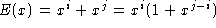Note: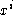is not divisible by G(x) if it contains two or more terms. Thus, we can detect double-bit errors if G(x) does not dividefor any k up to the message size.

Satisfactory generator polynomials can be found.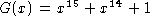, for instance, does not dividefor.

• odd number of bits.

• burst errors less than or equal to degree. Note: A polynomial with r check bits will detect all burst errors of length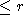.

What transmitted message will be an error but still generate a checksum of zero on receiving end? (T(x) + E(x))/G(x) so if E(x) = G(x).

### CRC Standards

There are currently three international standards:

• CRC-12:• CRC-16:• CRC-CCITT: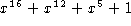Note: 16-bit CRCs detect all single and double errors, all errors with odd number of bits, all burst errors of length16 bits, and 99.997% of 17-bit errors.

Is usually done in hardware!

### MD5

Message-digest algorithm for compressing a large message.

Take a message and produce a 128-bit message digest. This compact form can be used to validate the received copy of the message.